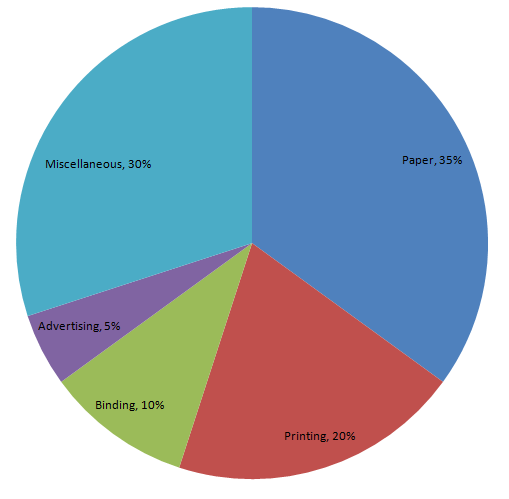# The following table shows the expenditure incurred by a publisher in publishing a book:

Question:

The following table shows the expenditure incurred by a publisher in publishing a book:

 Items Paper Printing Binding Advertising Miscellaneous Expenditure (in%) 35% 20% 10% 5% 30%

Present the above data in the form of a pie-chart.

Solution:

We know:

Central angle of a component = (component value/sum of component values ×">× 360)

Here the total % of expenditures = 100%

Thus the central angle for each component can be calculated as follows:

 Item Expenditure (in %) Sector angle Paper 35 35/100 ×">× 360 = 126 Printing 20 20/100 ×">× 360 = 72 Binding 10 10/100 ×">× 360 = 36 Advertising 5 5/100 ×">× 360 = 18 Miscellaneous 30 30/100 ×">× 360 = 108

Now, the pie chat representing the given data can be constructed by following the steps below:

Step 1 : Draw circle of an appropriate radius.

Step 2 : Draw a vertical radius of the circle drawn in step 1.

Step 3 : Choose the largest central angle. Here, the largest central angle is 126o. Draw a sector with the central angle 126o in such a way that one of its radii coincides with the radius drawn in step 2 and another radius is in its counter clockwise direction.

Step 4 : Construct other sectors representing other items in the clockwise direction in descending order of magnitudes of their central angles.

​Step 5 : Shade the sectors with different colours and label them, as shown as in figure below.​​